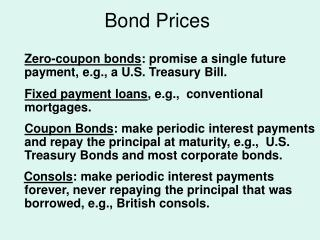DownloadDownload PresentationBond Prices

# Bond Prices

Télécharger la présentation## Bond Prices

- - - - - - - - - - - - - - - - - - - - - - - - - - - E N D - - - - - - - - - - - - - - - - - - - - - - - - - - -
##### Presentation Transcript

1. Bond Prices Zero-coupon bonds: promise a single future payment, e.g., a U.S. Treasury Bill. Fixed payment loans, e.g., conventional mortgages. Coupon Bonds: make periodic interest payments and repay the principal at maturity, e.g., U.S. Treasury Bonds and most corporate bonds. Consols: make periodic interest payments forever, never repaying the principal that was borrowed, e.g., British consols.

2. Bond Prices Zero-Coupon Bonds or Discount Bonds Price of a \$100 face value zero-coupon bond Where i = interest rate n = time until the payment is made

3. Bond Prices Zero-Coupon Bonds or Discount Bonds Examples. Assume i=4% Price of a One-Year Treasury Bill. Price of a Six-Month Treasury Bill

4. Bond Prices Fixed Payment Loans Value of a Fixed Payment Loan =

5. Bond Prices Coupon Bond

6. Bond Prices Consols

7. Bond Yields Yield to Maturity Price of One-Year 5 percent Coupon Bond = The value of i that solves this equation is the yield to maturity

8. Bond Yields Yield To Maturity • If the price of the bond is \$100, then the yield to maturity equals the coupon rate. • Since the price rises as the yield falls, when the price is above \$100, the yield to maturity must be below the coupon rate. • If you pay more than the face value for a bond, you are earning less than the coupon rate.

9. Bond Yields

10. Bond Yields

11. Bond Yields Holding Period Return: the return to holding a bond and selling it before maturity. The holding period return can differ from the yield to maturity when you do not hold the bond to maturity.

12. Bond Yields Example: 10 year bond (Face value=\$100) and a 6% coupon rate. Sold one year later. • If the interest rate in one year is 5%, one year holding period return =

13. Bond Yields If the interest rate in one year is 7% One year holding Period return = or -.52%

14. Bond Market and Interest Rates One Year Zero-coupon (discount) Bond.

15. Bond Market and Interest Rates

16. Bond Market and Interest Rates Factors that shift Bond Supply • An increase in the government’s borrowing increases the quantity of bonds outstanding, shifting the bond supply curve to the right. • As business conditions improve, the bond supply curve shifts to the right. • An increase in expected inflation shifts the bond supply curve to the right.

17. Bond Market and Interest Rates

18. Factors that Shift Bond Demand to Right • An increases in wealth. • A fall in expected inflation. • If the return on bonds rises relative to the return on alternative investments, the demand for bonds increases. • If a bond becomes less risky relative to alternative investments, the demand for the bond increases. • When a bond becomes more liquid relative to alternatives, the demand for the bond increases.

19. Bond Market and Interest Rates

20. Bonds and Risk Sources of Bond Risk • Default Risk • Inflation Risk • Interest-Rate Risk